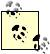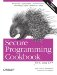# Multiplying Matricies

Problem

You want to perform efficient multiplication of two matricies.

Solution

Example 11-32 shows an implementation of matrix multiplication that can be used with both the dynamic- or fixed-size matrix implementations. This algorithm technically produces the result of the equation A=A+B*C, which is, perhaps surprisingly, an equation more efficiently computed than A=B*C.

Example 11-32. Matrix multiplication

```#include "matrix.hpp" // recipe 11.13
#include "kmatrix.hpp" // recipe 11.14
#include
#include

using namespace std;

template
void matrixMultiply(const M1& m1, const M2& m2, M3& m3)
{
assert(m1.cols( ) == m2.rows( ));
assert(m1.rows( ) == m3.rows( ));
assert(m2.cols( ) == m3.cols( ));
for (int i=m1.rows( )-1; i >= 0; --i) {
for (int j=m2.cols( )-1; j >= 0; --j) {
for (int k = m1.cols( )-1; k >= 0; --k) {
m3[i][j] += m1[i][k] * m2[k][j];
}
}
}
}

int main( )
{
matrix m1(2, 1);
matrix m2(1, 2);
kmatrix m3;
m3 = 0;
m1 = 1; m1 = 2;
m2 = 3; m2 = 4;
matrixMultiply(m1, m2, m3);
cout << "(" << m3 << ", " << m3 << ")" << endl;
cout << "(" << m3 << ", " << m3 << ")" << endl;
}```

Example 11-32 produces the following output:

```(3, 4)
(6, 8)```

Discussion

When multiplying two matricies, the number of columns in the first matrix must be equal to the number of rows in the second matrix. The resulting matrix has the number of rows of the first matrix and the number of columns of the second matrix. I assure that these conditions are true during debug builds by using the assert macro found in the header.

The key to efficient matrix multiplication is to avoid any superfluous creation and copying of temporaries. Thus, the matrix multiplication function in Example 11-32 was written to pass the result by reference. If I had written a straightforward multiplication algorithm by overriding operator* it would result in the overhead of an unneccessary allocation, copy, and deallocation of a temporary matrix. This can be potentially very expensive when dealing with large matricies.The reason the matrix multiplication equation in Example 11-32 computes A=A+B*C instead of A=B*C is that it avoids unneccessarily initializing the values of A.

Recipe 11.17Secure Programming Cookbook for C and C++: Recipes for Cryptography, Authentication, Input Validation & More
ISBN: 0596003943
EAN: 2147483647
Year: 2006
Pages: 241